## 含间隙铰接的柔性航天器刚柔耦合动力学与控制研究1)

*上海航天控制技术研究所,上海 201109

## DYNAMICS AND CONTROL OF RIGID-FLEXIBLE COUPLING FLEXIBLE SPACECRAFT WITH JOINT CLEARANCE1)

Sun Jie*,, Sun Jun,*,,2), Liu Fucheng*,, Zhu Dongfang*,, Huang Jing*,

*Shanghai Aerospace Control Technology Institute, Shanghai 201109,China

Shanghai Key Laboratory of Aerospace Intelligent Control Technology,Shanghai 201109,China

 基金资助: 1) 国家自然科学基金资助项目.  11772185国家自然科学基金资助项目.  61903245国家自然科学基金资助项目.  61803258

Received: 2020-04-14   Accepted: 2020-07-11   Online: 2020-11-18Abstract

There are still lots of joint clearances that cannot be eliminated for large-scale flexible spacecraft in post-lock phase. Joint clearance directly affects the attitude maneuver of the flexible spacecraft as well as the pointing accuracy and stability of the payload, which has a great influence on the dynamic characteristics of the spacecraft. Aiming at this issue, a dynamic modelling and control method for the rigid-flexible coupling spacecraft with joint clearance is proposed in this paper. The accurate dynamic model of the joint with clearance is established firstly, thus the dynamic model of flexible structure with joint clearances is built. Then the discrete rigid-flexible coupling nonlinear dynamic model of the spacecraft with clearances is obtained by Hamilton principle and modal discrete method. The Newmark algorithm is used to solve the nonlinear equation. Based on macro fiber composite (MFC) actuator, the rigid-flexible-electrical coupling dynamic equation of the spacecraft is obtained and the control law is designed by the optimum control. The influences of joint parameters, moment of inertia of central rigid body, clearance size and clearance number on the dynamic characteristics of the spacecraft are analyzed. The effects of joint clearance on the attitude maneuver and structural vibration of the spacecraft are emphatically studied. Finally, the active control is applied to the spacecraft using MFC actuator. The results reveal that the joint parameters and moment of inertia of the central rigid body affect the natural frequency of the spacecraft. With the increase of the size of joint clearance and number of clearances, the overall stiffness of the spacecraft decreases gradually, while the attitude angle and vibration displacement response of the spacecraft increase. Through the active control based on MFC, the cooperative control of the attitude maneuver and structural vibration of the spacecraft with clearance can be realized, and the effects of clearance on the dynamic characteristics of the spacecraft can be alleviated.

Keywords： flexible spacecraft ; joint clearance ; rigid-flexible coupling ; macro fiber composite ; vibration control

Sun Jie, Sun Jun, Liu Fucheng, Zhu Dongfang, Huang Jing. DYNAMICS AND CONTROL OF RIGID-FLEXIBLE COUPLING FLEXIBLE SPACECRAFT WITH JOINT CLEARANCE1). Chinese Journal of Theoretical and Applied Mechanics[J], 2020, 52(6): 1569-1580 DOI:10.6052/0459-1879-20-109

## 1 含间隙铰接结构模型

$F_{{a}j} = \left\{ \!\! \begin{array}{ll} 0 , & \theta _{s + 1} - \theta _s \leqslant d_j \\ k_j (\theta _{s + 1} - \theta _s - d_j ) , & \theta _{s + 1} - \theta _s > d_j \\ k_j (\theta _{s + 1} - \theta _s + d_j ) , & \theta _{s + 1} - \theta _s < - d_j \end{array} \!\! \right.$

### 图1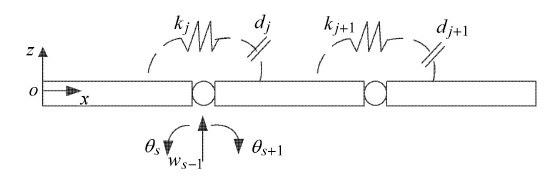Fig. 1   Schematic diagram of the flexible beam connected by joints with clearances

${\pmb M}_j \left( {a,b} \right) = \left\{ \begin{array}{ll} m_j , & a = h, b = h \\ 0 , & a \ne h, b \ne h \end{array} \right.$

${\pmb K}_j$ 是由铰链引入的结构刚度阵,可表示为

${\pmb K}_j \left( {a,b} \right) = \left\{\!\!\begin{array}{cl} k_j , & a = b = h1 , \ a = b = h2\\ - k_j , & a = h1 , \ b = h2 \ {or} \ a = h2 , \ b = h1 \\ 0 , & a \ne h1, h2 ; \ b \ne h1, h2 \end{array}\!\!\right.$

$\bar {\pmb M}\ddot {\pmb x} + \bar {\pmb C}\dot {\pmb x} + \bar {\pmb K} {\pmb x} = \bar {\pmb F}$

$\hat{\pmb M}\ddot{\pmb q} + \hat{\pmb C}\dot{\pmb q} + \hat{\pmb K}{\pmb q} = \hat {\pmb F} + \hat{\pmb F}_{h}$

$\left. \begin{array} \hat{\pmb M} ={\pmb \varPhi}^{T}\bar{\pmb M}{\pmb \varPhi} , \ \hat{\pmb C} = {\pmb \varPhi}^{T}\bar{\pmb C}{\pmb \varPhi} , \ \hat{\pmb K} = {\pmb \varPhi}^{T}\bar{\pmb K}{\pmb \varPhi} \\ \hat{\pmb F} = {\pmb \varPhi}^{T}{\pmb F} , \ \hat{\pmb F}_{h} ={\pmb \varPhi}^{T}{\pmb F}_{a}\end{array} \right\}$

## 2 刚-柔耦合非线性动力学模型建立

${\pmb r} = \left( {r_0 + x} \right){\pmb i} + w{\pmb k}$

### 图2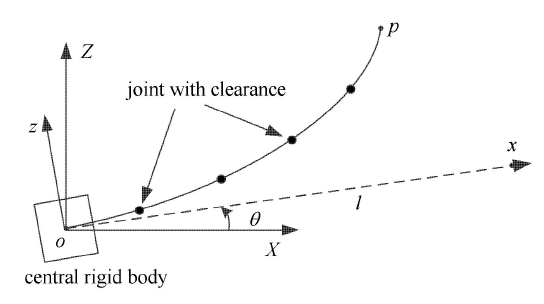Fig. 2   Schematic diagram of flexible spacecraft with clearance joints

${\pmb v} = \dot{\pmb r} + \dot {\theta }{\pmb k}\times {\pmb r}$

$T = \dfrac 12 \rho _1 \int_0^l {\pmb v \pmb v} \text{d} x + \dfrac 12 \dot {\theta }J_{1} \dot {\theta }$

$U = \dfrac 12 \int_0^l EI({w}'')^{2} \text{d} x +\sum_{j = 1}^{n_j } \dfrac{1}{2} k_j \left( {\theta _{j2} - \theta_{j1} } \right)^2$

$\int_{t_1 }^{t_2 } {\delta (T - U)} \text{d} t = 0$

$J\ddot {\theta } + \int_0^l {\rho _1 \left( {x + r_0 } \right)\ddot {w}} \text{d} x + \ddot {\theta }\rho _1 \int_0^l {w^2} \text{d} x +\\ \dot {\theta }\rho _1 \int_0^l {2w\dot {w}} \text{d} x = 0$
$EI{w}'''' + \rho _1 \left[ {\left( {x + r_0 } \right)\ddot {\theta } + \ddot {w}} \right] - \rho _1 \dot {\theta }^2w = 0$

$\left.\begin{array}{cc} {EI{w}'''\left( l \right) = 0 , \ \ EI{w}''\left( l \right) = 0} \\ {{w}'\left( 0 \right) = 0 , \ \ w\left( 0 \right) = 0} \end{array} \!\!\right\}$

$\left[\!\! \begin{array}{cc} J + {\pmb q}^{T}\hat {\pmb M}{\pmb q} & {\pmb H} \\ {\pmb H}^{T} & \hat {\pmb M} \end{array} \!\! \right]\left[\!\! \begin{array}{c} \ddot {\theta } \\ \ddot {\pmb q} \end{array} \!\! \right] + \left[\!\! \begin{array}{cc} 0 & {\bf 0} \\ {\bf 0} & \hat{\pmb C} \end{array} \!\! \right]\left[\!\! \begin{array}{c} \dot {\theta } \\ \dot{\pmb q} \end{array} \!\! \right] + \left[\!\! \begin{array}{cc} 0 & {\bf 0} \\ {\bf 0} & \hat{\pmb K} - \dot {\theta }^2\hat{\pmb M} \end{array} \!\! \right]\left[\!\! \begin{array}{c} \theta \\ {\pmb q } \end{array} \!\! \right] = \left[\!\! \begin{array}{c} - 2\dot {\theta }{\pmb q}^{T}\hat {\pmb M}\dot {\pmb q} \\ \hat {\pmb F} + \hat{\pmb F}_{h } \end{array} \!\! \right]$

## 3 数值算法和控制算法

$\tilde {\pmb M}\left( {0} \right) = \left[\!\! \begin{array}{cc} J + {\pmb q}_0^{T}\hat{\pmb M}{\pmb q}_0 & {\pmb H} \\ {\pmb H}^{T} & \hat{\pmb M} \end{array} \!\! \right] , \ \ \tilde{\pmb C}\left( {0} \right) = \left[\!\! \begin{array}{cc} {\bf 0} & {\bf 0} \\ {\bf 0} & \hat{\pmb C} \end{array} \!\! \right]\\ \tilde{\pmb K}\left( {0} \right) = \left[\!\! \begin{array}{cc} {\bf 0} & {\bf 0} \\ {\bf 0} & \hat{\pmb K} - \dot {\theta }_0^2 \hat{\pmb M} \end{array} \!\! \right] , \ \ \bar{\pmb q} = \left[\!\! \begin{array}{c} \theta \\ {\pmb q} \end{array} \!\! \right]$

$\ddot {\bar {\pmb q}}_0 = \tilde{\pmb M}^{ - 1}\left( 0 \right) \left( {\tilde {\pmb F}\left( 0 \right) - \tilde{\pmb K}\left( 0 \right)\bar{\pmb q}_0 - \tilde {\pmb C}\left( 0 \right)\dot {\bar{\pmb q}}_0 } \right)$

(1) 求解第$i$个时间步长的广义位移 $\bar{\pmb q}\left( {i + {1}}\right)$、广义加速度 $\ddot {\bar{\pmb q}}\left( {i + {1}}\right)$ 和广义速度 $\dot {\bar{\pmb q}}\left( {i + {1}} \right)$;

(2) 根据公式 (1) 判断第 $( i +1)$ 个时间步长铰链碰撞是否发生;

(3) 利用 $\bar{\pmb q}\left( {i + {1}} \right)$ 和 $\dot {\bar{\pmb q}}\left( {i + {1}} \right)$ 计算第 $( i +1)$ 个时间步长的非线性质量矩阵 $\tilde{\pmb M}\left( {i + {1}} \right)$、非线性刚度矩阵 $\tilde{\pmb K}\left( {i + {1}}\right)$ 及广义力矩阵 $\tilde{\pmb F}\left( {i + {1}} \right)$：

$\tilde {\pmb M}\left( {i + 1} \right) = \left[\!\! \begin{array}{cc} {J + {\pmb q}^{T}\left( {i + 1} \right)\hat{\pmb M}{\pmb q}\left( {i + 1} \right)} & {\pmb H }\\ {\pmb H}^{T} & \hat{\pmb M} \end{array} \!\! \right]$
$\tilde{\pmb C}\left( {i + 1} \right) = \left[\!\! \begin{array}{cc} 0 & {\bf 0} \\ {\bf 0} & \hat{\pmb C} \end{array} \!\! \right]$
$\tilde{\pmb K}\left( {i + 1} \right) = \left[\!\! \begin{array}{cc} 0 & {\bf 0} \\ {\bf 0} & {\hat{\pmb K} - \dot {\theta }^2\left( {i + 1} \right)\hat {\pmb M}} \end{array} \!\! \right]$
$\tilde{\pmb F}\left( {i + 1} \right) = \left[\!\! \begin{array}{c} { - 2\dot {\theta }\left( {i + 1} \right) {\pmb q}^{T}\left( {i + 1} \right)\hat{\pmb M}\dot{\pmb q}\left( {i + 1} \right)} \\ {\hat{\pmb F}\left( {i + 1} \right) + \hat{\pmb F}_{h} \left( {i + 1} \right)} \\ \end{array} \!\! \right]$

$\left[ \!\! \begin{array}{cc} J & {\pmb H} \\ {\pmb H}^{T} & \hat {\pmb M} \end{array} \!\! \right]\left[\!\! \begin{array}{c} \ddot {\theta } \\ \ddot{\pmb q} \end{array} \!\! \right] + \left[\!\! \begin{array}{cc} 0 & {\bf 0} \\ {\bf 0} & \hat{\pmb C} \end{array}\!\! \right]\left[\!\! \begin{array}{c} \dot {\theta } \\ \dot {\pmb q} \end{array}\!\! \right] + \left[\!\! \begin{array}{cc} 0 & {\bf 0} \\ {\bf 0} & \hat{\pmb K} \end{array} \!\! \right]\left[\!\! \begin{array}{c} \theta \\ {\pmb q} \end{array} \!\! \right] = \\ \left[\!\! \begin{array}{c} 0 \\ {\hat {\pmb F} + \hat{\pmb F}_{h} + \hat{\pmb F}_{p} } \end{array}\!\! \right]$

${\pmb M}_{s} \ddot {\bar{\pmb q}} +{\pmb C}_{s} \dot {\bar{\pmb q}} + {\pmb K}_{s} \bar{\pmb q} = \left[ \!\! \begin{array}{c} 0 \\ {\pmb \varPhi}^{T}{\pmb K}_{u\varphi } {\pmb U} \end{array} \!\! \right] + \left[\!\! \begin{array}{c} 0 \\ \hat {\pmb F} + \hat{\pmb F}_{h} \end{array} \!\! \right]$

$\dot {\pmb Z} = {\pmb A}{\pmb Z} + {\pmb B}{\pmb U} + \left[ \!\! \begin{array}{c} 0 \\ {\pmb M}_\text{s}{s}^{ - 1} [0 \ \ \hat{\pmb F} + \hat{\pmb F}_{h} ]^{T} \end{array} \!\! \right]$

${\pmb Z}=\left[\!\! \begin{array}{c} \bar{\pmb q} \\ \dot{\bar{\pmb q}} \end{array} \right] , \ \ \ {\pmb A}=\left [\!\! \begin{array}{cc} {\bf 0} & {\pmb I} \\ -{\pmb M}^{-1}_{s} {\pmb K}_{s} & -{\pmb M}^{-1}_{s} {\pmb C}_{s} \end{array} \right]$

${\pmb B}=\left[\!\! \begin{array}{c} {\bf 0} \\ {\pmb M}^{-1}_{s} [ 0 \ \ {\pmb \varPhi}^{T} {\pmb K}_{u\varphi} ]^{T} \end{array} \right]$

$J = \dfrac{1}{2}\int_0^\infty \left( {{\pmb Z}^{T}{\pmb Q}{\pmb Z} + {\pmb U}^{T}{\pmb R}{\pmb U}} \right)\text{d} t$

## 4 数值模拟与讨论

### 4.1 模型验证

Table 1  Comparison of natural frequencies of the two models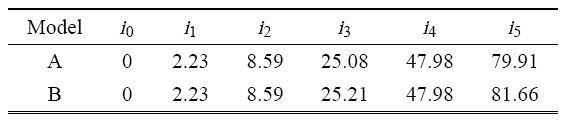### 图3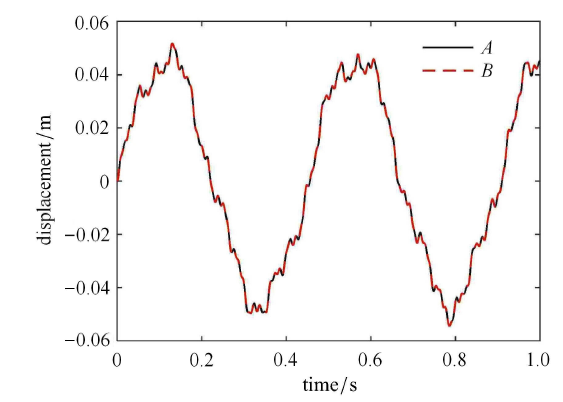Fig. 3   Comparison of vibration displacement of the two models

### 图4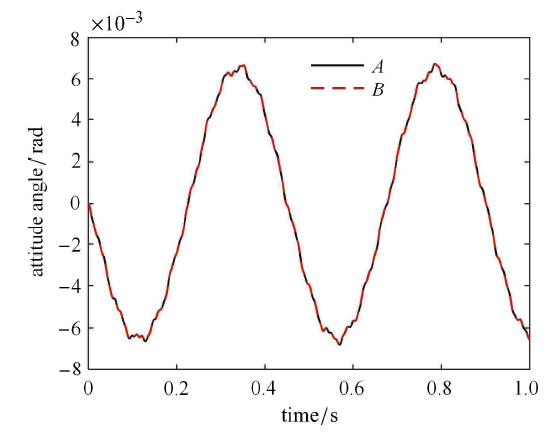Fig. 4   Comparison of attitude angle of spacecraft of the two models

### 4.2 铰链参数及中心刚体转动惯量对航天器动力学特性的影响

Table 2  Natural frequencies of the spacecraft with different joint masses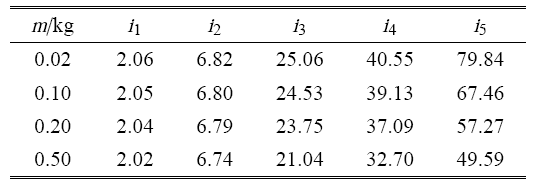Table 3  Natural frequencies of the spacecraft with different moment of inertia of central rigid body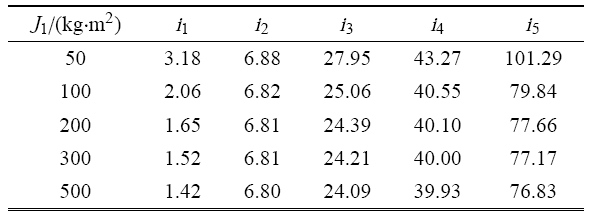Table 4  Percentage of the natural frequency of the spacecraft with different joint stiffness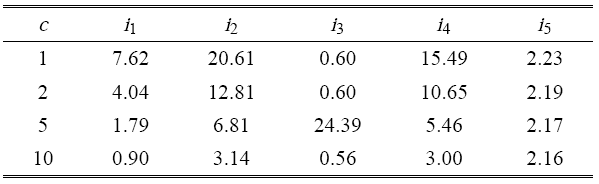### 图5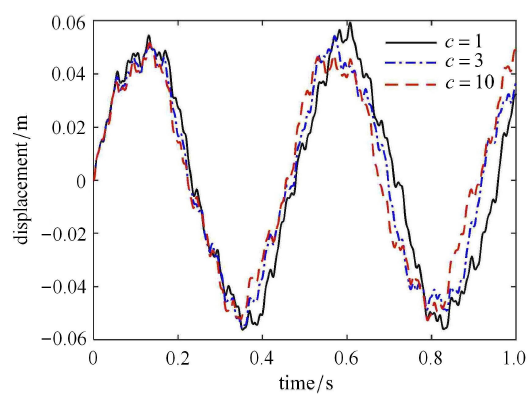Fig. 5   Vibration displacements of the flexible body with different joint connection stiffness

### 图6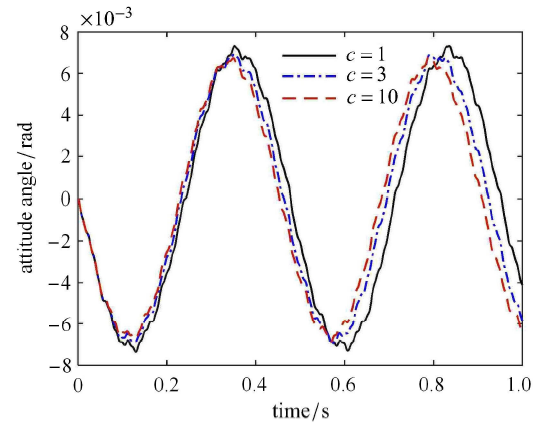Fig. 6   Attitude angle of the spacecraft with different joint connection stiffness

### 图7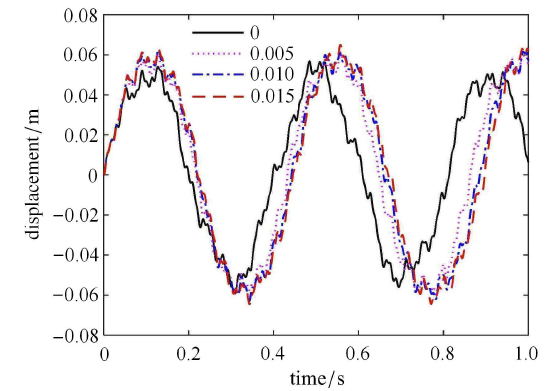Fig. 7   Vibration displacements of the flexible body with different clearance sizes

### 图8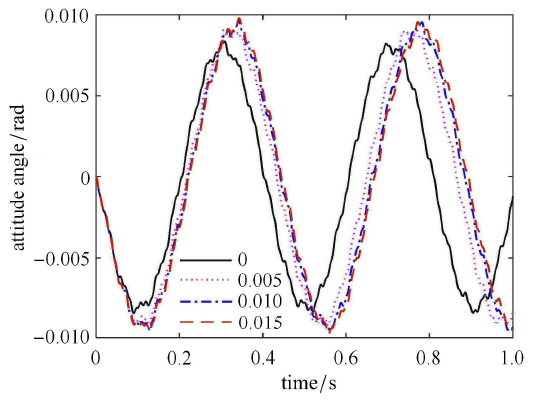Fig. 8   Attitude angle of the spacecraft with different clearance sizes

### 图9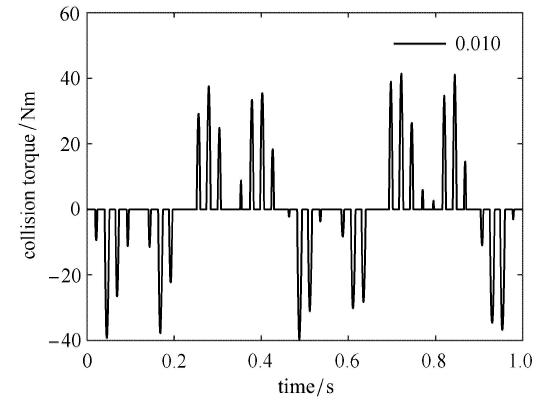Fig. 9   Collision torque of the joint with clearance 0.01 rad

### 图10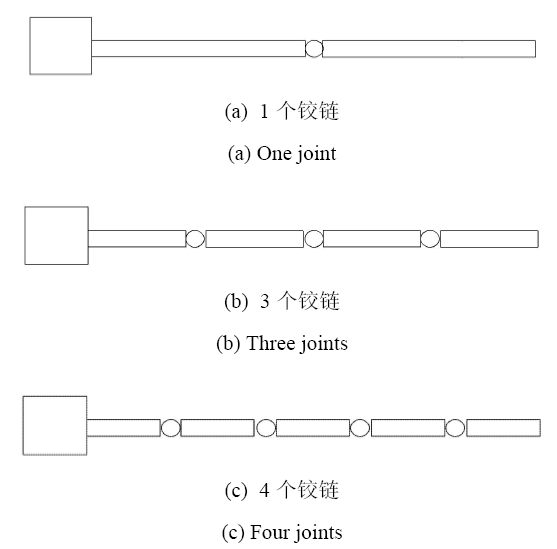Fig. 10   Diagram of spacecraft with different number of joints

Table 5  The natural frequencies of spacecraft with different number of Joints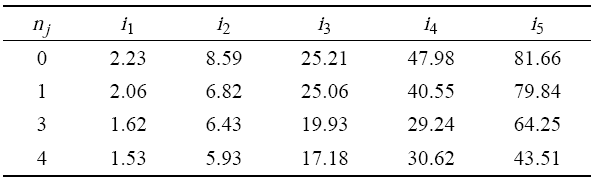### 图11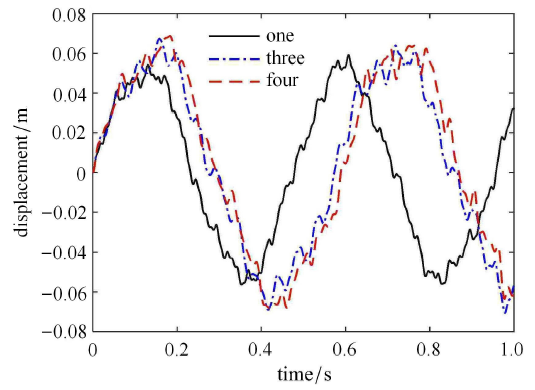Fig. 11   Vibration displacements of the flexible body with different number of joints

### 图12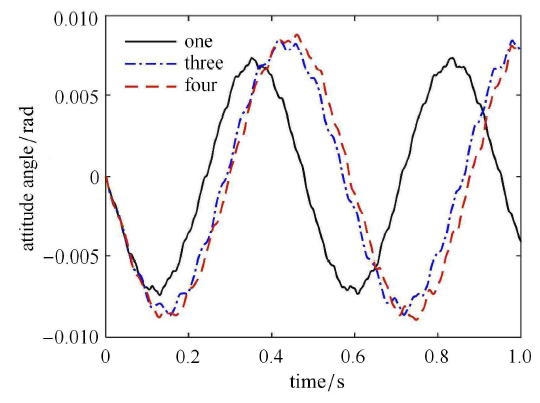Fig. 12   Attitude angle of the spacecraft with different number of joints

### 图13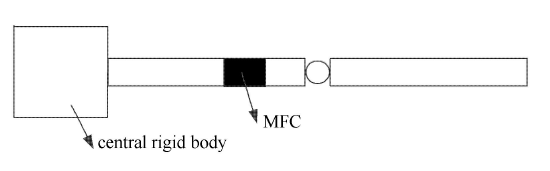Fig. 13   Schematic of spacecraft with clearance joints with MFC actuator

### 图14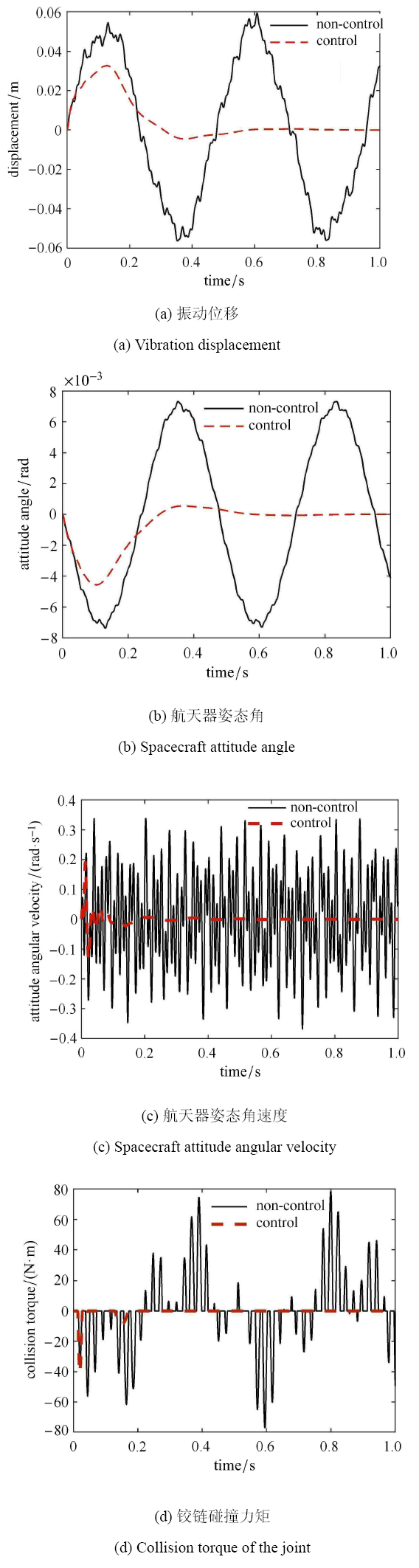Fig. 14   Comparison of dynamic characteristics of the spacecraft system between non-control and control models

### 图15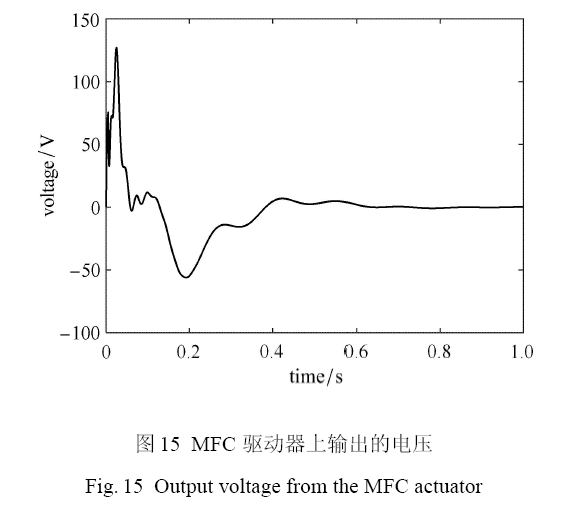Fig. 15   Output voltage from the MFC actuator

## 参考文献 原文顺序 文献年度倒序 文中引用次数倒序 被引期刊影响因子

( Hu Haiyan, Tian Qiang, Zhang Wei, et al.

Nonlinear dynamics and control of large deployable space structures composed of trusses and meshes

Advances in Mechanics, 2013,43(4):390-414 (in Chinese))

Moon FC, Li GX.

Experimental study of chaotic vibrations in a pin-jointed space truss structure

AIAA Journal, 1990,28:915-921

Bendiksen OO.

Mode localization phenomena in large space structures

AIAA Journal, 1987,25:1241-1248

( Cao Dengqing, Chu Shiming, Li Zhengfa, et al.

Study on the non-smooth mechanical models and dynamics for space deployable mechanisms

Chinese Journal of Theoretical and Applied Mechanics, 2013,45(1):3-15 (in Chinese))

( Gao Chentong, Li Liang, Zhang Dingguo, et al.

Dynamic modeling and simulation of rotating FGM tapered beams with shear effect

Chinese Journal of Theoretical and Applied Mechanics, 2018,50(3):654-666 (in Chinese))

( Wu Ji, Zhang Dingguo, Li Liang, et al.

Dynamic characteristics analysis of a rotating flexible curved beam with a concentrated mass

Chinese Journal of Theoretical and Applied Mechanics, 2019,51(4):1134-1147 (in Chinese))

( Cao Dengqing, Bai Kunchao, Ding Hu, et al.

Advances in dynamics and vibration control of large-scale flexible spacecraft

Chinese Journal of Theoretical and Applied Mechanics, 2019,51(1):1-13 (in Chinese))

URL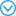( Yan Shaoze, Xiang Wuweikai, Huang Tieqiu.

Advances in modeling of clearance joints and dynamics of mechanical systems with clearances

Acta Scientiarum Naturalium Universitatis Pekinensis, 2016,52(4):741-755 (in Chinese))

URLRecent developments in modeling of clearance joints and dynamics of mechanical systems with clearances are reviewed. Different modeling approaches for clearance joints are summarized firstly, which comprise the massless link approach, the non-smooth dynamics approach, the contact force approach and the 3D revolute joint approach. Then, applications of these approaches in the study of the nonlinear dynamics, and performance and reliability evaluation of the mechanical systems with clearances are systematically reviewed. Finally, the key problems and priorities which need to be further studied are proposed, including the modeling of clearace joints considering stick-slip phenomenon, contact surface profile and conformal contact condition, the dynamic analysis and kinematic accuracy evaluation of mechanical systems with both clearances and uncertainties, and the design for clearance joints.

Marques F, Isaac F, Dourado N, et al.

An enhanced formulation to model spatial revolute joints with radial and axial clearances

Mechanism and Machine Theory, 2017,116:123-144

Cavalieri FJ, Cardona A.

Non-smooth model of a frictionless and dry three-dimensional revolute joint with clearance for multibody system dynamics

Mechanism and Machine Theory, 2018,121:335-354

Tian Q, Flores P, Lankarani HM.

A comprehensive survey of the analytical, numerical and experimental methodologies for dynamics of multibody mechanical systems with clearance or imperfect joints

Mechanism and Machine Theory, 2018,122:1-57

Zheng XD, Zhang F, Wang Q.

Modeling and simulation of planar multibody systems with revolute clearance joints considering stiction based on an LCP method

Mechanism and Machine Theory, 2018,130:184-202

Akhadkar N, Acary V, Brogliato B.

Multibody systems with 3D revolute joints with clearances: An industrial case study with an experimental validation

Multibody System Dynamics, 2018,42:249-282

Guo JN, He P, Liu ZS, et al.

Investigation of an improved planar revolute clearance joint contact model with rough surface

Tribology International, 2019,134:385-393

Zhao QQ, Guo JK, Hong J, et al.

Analysis of angular errors of the planar multi-closed-loop deployable mechanism with link deviations and revolute joint clearances

Aerospace Science and Technology, 2019,87, 25-36

Wang XP, Liu G, Ma SJ, et al.

Study on dynamic responses of planar multibody systems with dry revolute clearance joint: numerical and experimental approaches

Journal of Sound and Vibration, 2019,438:116-138

Xiang WWK, Yan SZ.

Dynamic analysis of space robot manipulator considering clearance joint and parameter uncertainty: Modeling, analysis and quantification

Acta Astronautica, 2020,169:158-169

Xiang WWK, Yan SZ, Wu JN, et al.

Dynamic response and sensitivity analysis for mechanical systems with clearance joints and parameter uncertainties using Chebyshev polynomials method

Mechanical Systems and Signal Processing, 2020,138:106596

Qian MB, Qin Z, Yan SZ, et al.

A comprehensive method for the contact detection of a translational clearance joint and dynamic response after its application in a crank-slider mechanism

Mechanism and Machine Theory, 2020,145:103717

( Wang Wei, Yu Dengyun, Ma Xingrui.

Advances and trends of non-linear dynamics of space joint-dominated structure

Advances in Mechanics, 2006,36(2):233-238 (in Chinese))

Yoshida T.

Dynamic characteristic formulations for jointed space structures

Journal of Spacecraft and Rockets, 2006,43(4):771-779

Li TJ, Guo J, Cao YY.

Dynamic characteristics analysis of deployable space structures considering joint clearance

Acta Astronautica, 2011,68:974-983

( Zhang Jing, Guo Hongwei, Liu Rongqiang, et al.

Influence analysis of joints on nonlinear dynamic characteristics of articulated structures

Acta Aeronautica et Astronautica Sinica, 2014,35(5):1433-1445 (in Chinese))

Yao XG, Guo XS, Feng YB, et al.

Dynamic analysis for planar beam with clearance joint

Journal of Sound and Vibration, 2015,339:324-341

Wang Y, Li FM.

Nonlinear dynamics modeling and analysis of two rods connected by a joint with clearance

Applied Mathematical Modelling, 2015,39(9):2518-2527

Zhang J, Guo HW, Liu RQ, et al.

Nonlinear dynamic characteristic analysis of jointed beam with clearance

Acta Astronautica, 2016,129:135-146

Li B, Han LF, Wei J, et al.

Theoretical and experimental identification of cantilever beam with clearances using statistical and subspace-based methods

Journal of Computational and Nonlinear Dynamics, 2016,11:031003

Liu J, Li B.

Theoretical and experimental identification of clearance nonlinearities for a continuum structure

Journal of Computational and Nonlinear Dynamics, 2016,11:041019

( Xi An, Zhang Wei, Liu Hongli.

Analysis and experiment on dynamic stiffness of clearance hinge in large circular truss antenna

Scientia Sinica Physica, Mechanica & Astronomica, 2017,47(10):104606 (in Chinese))

Krysko VA, Awrejcewicz J, Papkova IV, et al.

On reliability of chaotic dynamics of two Euler-Bernoulli beams with a small clearance

International Journal of Non-linear Mechanics, 2018,104:8-18

Jiang GQ, Yang XD, Zhang W, et al.

The modeling and dynamic analysis of two jointed beams with clearance

Applied Mathematical Modelling, 2019,74, 528-539

( He Haonan, Yu Kaiping, Tang Hong, et al.

Vibration experiment and nonlinear modelling research on the folding fin with freeplay

Chinese Journal of Theoretical and Applied Mechanics, 2019,51(5):1476-1488 (in Chinese))

Hu BB, Liu J, Liu SH, et al.

Simultaneous multi-parameter identification algorithm for clearance-type nonlinearity

Mechanical Systems and Signal Processing, 2020,139:106423

( Bai Zhengfeng, Zhao Yang, Tian Hao.

Dynamics simulation of deployment for solar panels with hinge clearance

Journal of Harbin Institute of Technology, 2009,41(3):11-14 (in Chinese))

Bai ZF, Liu YQ, Sun Yi.

Investigation on dynamic responses of dual-axis positioning mechanism for satellite antenna considering joint clearance

Journal of Mechanical Science and Technology, 2015,29(2):453-460

Li YY, Wang ZL, Wang C, et al.

Planar rigid-flexible coupling spacecraft modeling and control considering solar array deployment and joint clearance

Acta Astronautica, 2018,142:138-151

( Li Min, Chen Weimin, Wang Mingchun, et al.

A load simulation method of piezoelectric actuator in FEM for smart structures

Science in China Series E: Technological Science, 2009,52(9):2576-2584 (in Chinese))

/

 〈〉